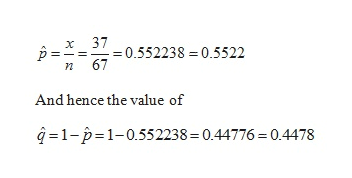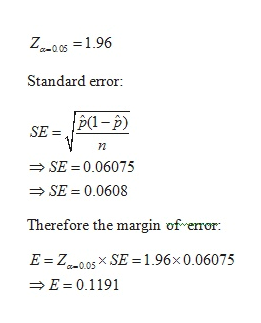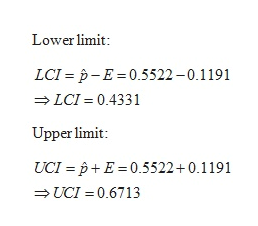For this problem, carry at least four digits after the decimal in your calculations. Answers may vary slightly due to rounding.In a random sample of 67 professional actors, it was found that 37 were extroverts.(a)Let p represent the proportion of all actors who are extroverts. Find point estimates for pand q. (Round your answer to four decimal places.)p̂=q̂=(b)Find a 95% confidence interval for p. (Round your answers to two decimal places.)Find the maximal margin of error. (Round your answer to two decimal places.)E = Report the bounds from the 95% confidence interval for p. (Round your answers to two decimal places.)lower limitupper limitGive a brief interpretation of the meaning of the confidence interval you have found.We are 95% confident that the true proportion of actors who are extroverts falls within this interval.We are 5% confident that the true proportion of actors who are extroverts falls above this interval.    We are 5% confident that the true proportion of actors who are extroverts falls within this interval.We are 95% confident that the true proportion of actors who are extroverts falls outside this interval.(c)Do you think the conditions np > 5 and nq > 5 are satisfied in this problem? Explain why this would be an important consideration.Yes, the conditions are satisfied. This is important because it allows us to say that p̂ is approximately normal.Yes, the conditions are satisfied. This is important because it allows us to say that p̂ is approximately binomial.    No, the conditions are not satisfied. This is important because it allows us to say that p̂ is approximately normal.No, the conditions are not satisfied. This is important because it allows us to say that p̂ is approximately binomial.

Question
For this problem, carry at least four digits after the decimal in your calculations. Answers may vary slightly due to rounding.
In a random sample of 67 professional actors, it was found that 37 were extroverts.
(a)
Let p represent the proportion of all actors who are extroverts. Find point estimates for pand q. (Round your answer to four decimal places.)
p̂=q̂=
(b)
Find a 95% confidence interval for p. (Round your answers to two decimal places.)
Find the maximal margin of error. (Round your answer to two decimal places.)
E =
Report the bounds from the 95% confidence interval for p. (Round your answers to two decimal places.)
lower limitupper limit
Give a brief interpretation of the meaning of the confidence interval you have found.
We are 95% confident that the true proportion of actors who are extroverts falls within this interval.We are 5% confident that the true proportion of actors who are extroverts falls above this interval.    We are 5% confident that the true proportion of actors who are extroverts falls within this interval.We are 95% confident that the true proportion of actors who are extroverts falls outside this interval.
(c)
Do you think the conditions np > 5 and nq > 5 are satisfied in this problem? Explain why this would be an important consideration.
Yes, the conditions are satisfied. This is important because it allows us to say that p̂ is approximately normal.Yes, the conditions are satisfied. This is important because it allows us to say that p̂ is approximately binomial.    No, the conditions are not satisfied. This is important because it allows us to say that p̂ is approximately normal.No, the conditions are not satisfied. This is important because it allows us to say that p̂ is approximately binomial.
Step 1

According to the given question,

In a random sample of 67 professional actors, it was found that 37 were extroverts.

Therefore the required sample proportion is determined as:help_outlineImage Transcriptionclose37 X 0.552238 0.5522 67 And hence the value of q1-p 1-0.552238 = 0.44776 = 0.4478 fullscreen
Step 2

Now the standard error and margin of error at 95% confidence interval that is at α = 0.05 level of significance is determine as:

Now the z critical value is determined as:help_outlineImage TranscriptioncloseZ-0s1.96 Standard error {p(1-p) SE=P- SE = 0.06075 SE = 0.0608 Therefore the margin of eror E Z005xSE = 1.96x 0.06075 E 0.1191 fullscreen
Step 3

Therefore the 95% confidence in...help_outlineImage TranscriptioncloseLower limit LCI p-E 0.5522-0.1191 LCI 0.4331 Upper limit: UCI pE 0.5522 + 0.1191 UCI 0.6713 fullscreen

Want to see the full answer?

See Solution

Want to see this answer and more?

Our solutions are written by experts, many with advanced degrees, and available 24/7

See Solution
Tagged in

Basic Probability